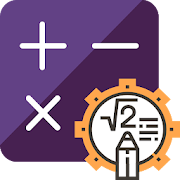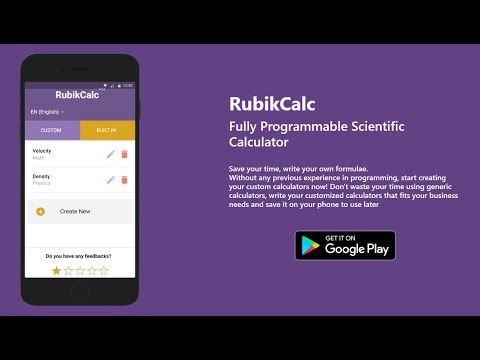# RubikCalcPRO: Programmable Calculator (PRO)Everyone
141RubikCalcPRO is a unique, fully programmable calculator that allows you to add all your most used functions at the swipe of a screen, having a very user friendly interface so just about anyone can program this calculator to be a physical, scientific or mathematical in one single custom formula calculator.

You can use your custom formula converted into calculator in no time without any programming skills or experience, just math.

Free Version Features:
2- See the results in real-time while you type.
4- Save your calculators to use them later
5- Supports Trigenometric functions (Sin,Cos,Tan ... )
6- Supports Logarithmic functions
7- Detailed Documentation

PRO Features
* See the steps of the solution step by step
* Table Mode: you can enter multiple inputs and get the output.
* Chart Mode: You can create customizable charts and share the results
* Share the results of each calculation.
* Customize your settings: Change the precision of the output (decimal places)

Use the following formula and see the results immediately in real-time debugging.

Example1 (Velocity Calculator)
Distance[m] = x
Time[s] = t
Velocity[m/s] = x/t

Example2 (Desnity Calculator):
Mass[kg]=m
Volume[m3] =v
Density[kg/m3] =m/v

Example3 (Volume Calculator):
Length=x
Width=y
Height=z
Volume=x*y*z
BaseArea=x*y

Example4 (Trigenometric Calculator):
Degrees=d

FAQs:
=====
* What is the "programmable custom formula Calculator"?
- It's an app that allows you to write your custom formula and convert it to custom calculator easily.

* How RubikCalc is different from any other programmable calculator?
- RubikCalc is totally different from other programmable calculator so that it's not a static app, it's totally dynamic, you can create your own customized formula calculators and save it and use it later.

* Can I use variables names like this p1,a3?
- No, you cannot mix numbers with letters in variable names, just use single symbols a,b,c,x,y,

* How does the app distinguishes between inputs and outputs?
- Outputs contains formula like this: Velocity=x/t,Square=x^2
While Inputs are just definitions like this: Distance=x, Time=t, Radius=r

* How can i specify units?
- Put the units between brackets after the name like this, Distance[m]=x

* How can i use the logarithmic function?
- Just write log(3),log(4).

* Can i use PI ?
- Yes, just write PI, all capital.
Collapse

Review Policy
4.3
141 total
5
4
3
2
1

## What's New

* Adding Ternary Operator, Check this documentation https://www.w3schools.com/js/js_comparisons.asp
* Show/Hide solution steps
Collapse

Eligible for Family LibraryEligible if bought after 7/2/2016. Learn More
Updated
August 8, 2019
Size
7.8M
Installs
10,000+
Current Version
2.5
Requires Android
4.4 and up
Content Rating
Everyone
Permissions
Offered By
Wisebay Solutions
Developer